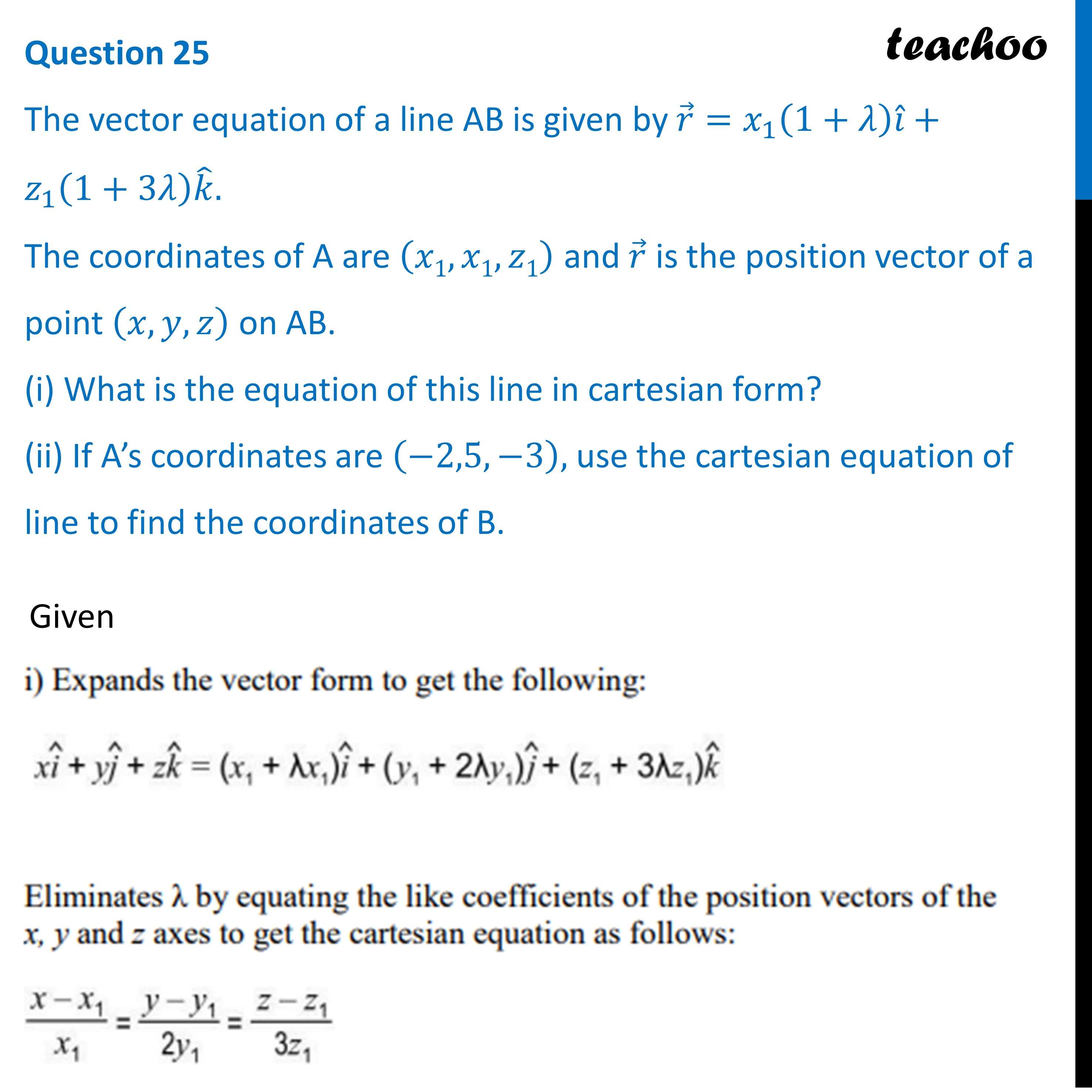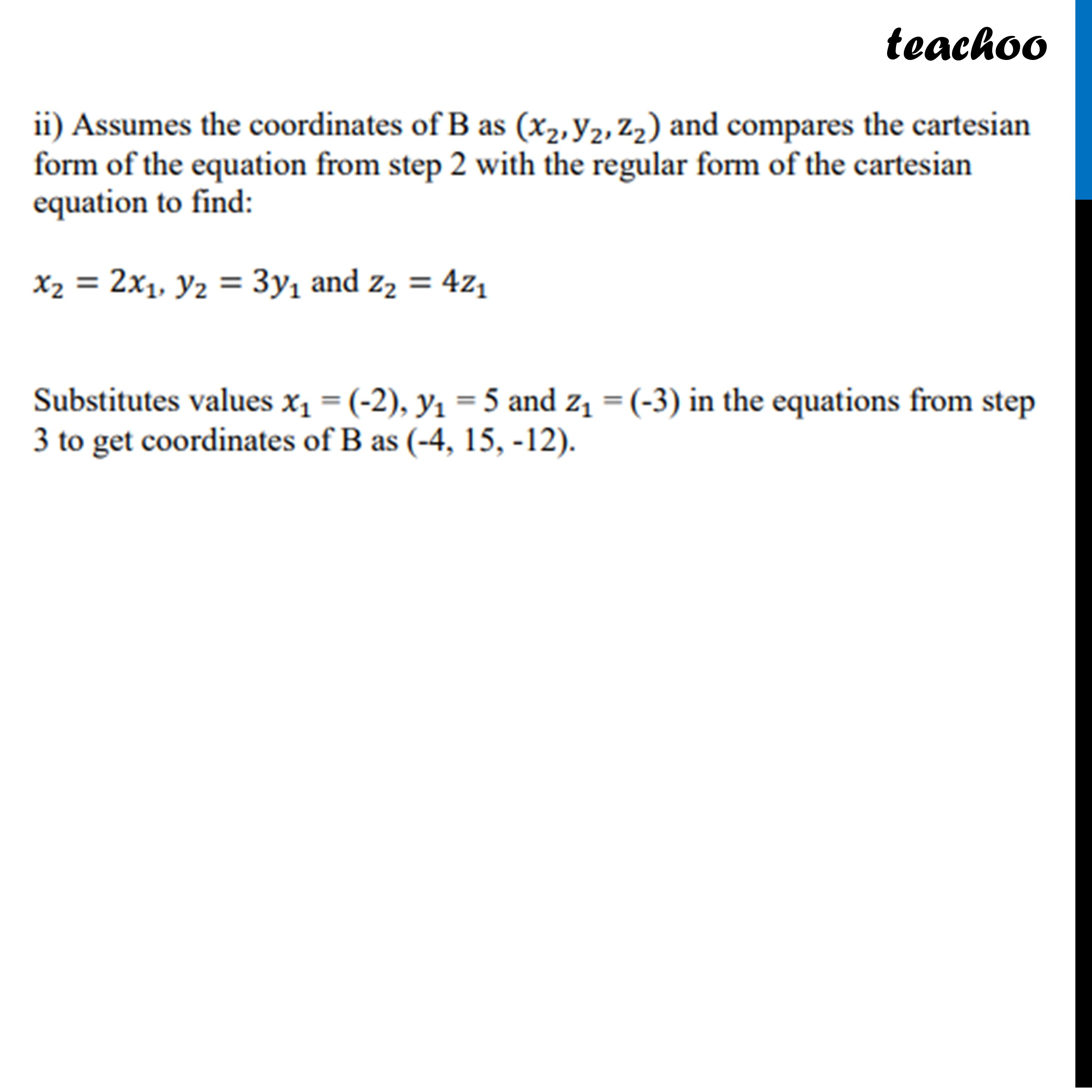Practice Questions CBSE - Maths Class 12 (2023 Boards)

Class 12
Solutions of Sample Papers and Past Year Papers - for Class 12 Boards

## (ii) If A’s coordinates are (-2,5,-3), use the cartesian equation of line to find the coordinates of B.Learn in your speed, with individual attention - Teachoo Maths 1-on-1 Class

### Transcript

The vector equation of a line AB is given by 𝑟 ⃗=𝑥_1 (1+𝜆) 𝑖 ̂+𝑧_1 (1+3𝜆) 𝑘 ̂. The coordinates of A are (𝑥1,𝑥1,𝑧1) and 𝑟 ⃗ is the position vector of a point (𝑥,𝑦,𝑧) on AB. (i) What is the equation of this line in cartesian form? (ii) If A’s coordinates are (−2,5,−3), use the cartesian equation of line to find the coordinates of B.